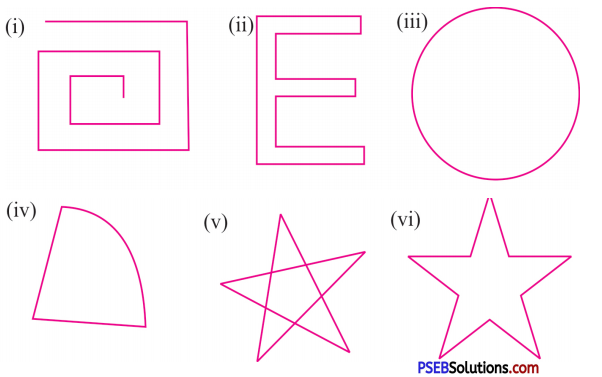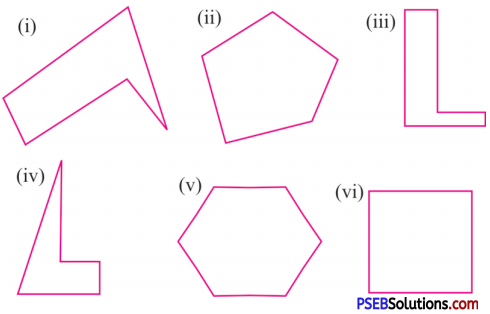# PSEB 6th Class Maths Solutions Chapter 9 Understanding Elementary Shapes Ex 9.5

Punjab State Board PSEB 6th Class Maths Book Solutions Chapter 9 Understanding Elementary Shapes Ex 9.5 Textbook Exercise Questions and Answers.

## PSEB Solutions for Class 6 Maths Chapter 9 Understanding Elementary Shapes Ex 9.5

1. Which of the following are polygons and there is no polygon. Give the reason:Solution:
(i) It is not a closed figure. Therefore it is not a polygon.
(ii) It is made up of lines segment. Therefore it is polygon.
(iii) It is not a polygon, because it is not made of line segments.
(iv) It is not closed by line segment. Therefore, it is not a polygon.
(v) It is not polygon because line segments are intersecting each other.
(vi) It is made up of line segments, therefore it is a polygon.2. Classify the following as concave or convex polygons:Solution:
(i) Concave Polygon
(ii) Convex Polygon
(iii) Concave Polygon
(iv) Concave Polygon
(v) Convex Polygon
(vi) Convex Polygon.

3. Tick in the boxes, if the property holds true for a particular quadrilateral otherwise eroes out ‘x’:

 Quadrilateral Properties Rectangle Parallelogram Rhombus Trapezium Square All sides are equal Only opposite sides are equal Diagonals are equal Diagonals bisect each other Diagonals are perpendicular to each other Each angle is 90°

Solution:

 Quadrilateral Properties Rectangle Parallelogram Rhombus Trapezium Square All sides are equal × × √ × √ Only opposite sides are equal √ √ × × × Diagonals are equal √ × × × √ Diagonals bisect each other √ √ √ × √ Diagonals are perpendicular to each other × × √ × √ Each angle is 90° √ × X × √4. Fill in the blanks:

Question (i)
…………… is a quadrilateral with only one pair of opposite sides parallel.
Solution:
Trapezium

Question (ii)
…………….. is a quadrilateral with all sides equal and diagonals of equal length.
Solution:
Square

Question (iii)
A polygon with atleast one angle is reflex is called ……………….. .
Solution:
Concave polygon

Question (iv)
Solution:
Square

Question (v)
…………… is a quadrilateral with opposite sides equal and diagonals of unequal length.
Solution:
Parallelogram.5. State True or False:

Question (i)
A rectangle is always a rhombus.
Solution:
False

Question (ii)
The diagonals of a rectangle are perpendicular to each other.
Solution:
False

Question (iii)
A square is a parallelogram.
Solution:
True

Question (iv)
A trapezium is a parallelogram.
Solution:
FalseQuestion (v)
Opposite sides of a parallelogram are parallel.
Solution:
True.